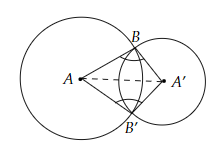# Prove that the line of centres of two intersecting circles subtends equal angles at the two points of intersection.

To do:

We have to prove that the line of centres of two intersecting circles subtends equal angles at the two points of intersection.

Solution:Let two circles with centres $A$ and $A'$ intersect each other at $B$ and $B'$ respectively.

In $\triangle BAA’$ and $\triangle B'AA’$

$AB = AB'$          (Radii of circle with centre $A$)

$A’B = A’B'$          (Radii of circle with centre $A'$)

$AA’ = AA’$         (Common side)

Therefore, by $SSS$ congruency,

$\triangle BAA’ \cong \triangle B'AA’$

This implies,

$\angle ABA' = \angle AB'A’$

From above,

The line of centres of two intersecting circles subtends equal angles at the two points of intersection.

Hence proved.

Updated on: 10-Oct-2022

40 Views